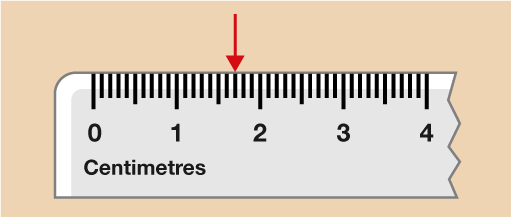Science, Maths & Technology

### Become an OU studentEveryday maths 1 (Wales)

Start this free course now. Just create an account and sign in. Enrol and complete the course for a free statement of participation or digital badge if available.

# 1.3 Measuring in millimetres and centimetres

When you’re measuring an item, you need to decide whether to measure it in millimetres, centimetres or metres. Often, your decision will be based on the size of the item, why you are measuring it and how accurate you need the measurement to be.

When you measure an item, you can actually express the same measurement in different ways. To help you to do this, you need to know some metric measure facts:

## Facts

10 millimetres = 1 centimetre

100 centimetres = 1 metre

1 000 metres = 1 kilometre

Starting with the smallest, metric units of length are millimetres (mm), centimetres (cm) and metres (m).

Kilometres (km) are used to measure distance.

## Example: Writing measurements in millimetres and centimetres

You can express a measurement in millimetres, centimetres or a combination of both. Look at the ruler below. What measurement is the arrow pointing to?Figure 8 Measuring a pen

### Method

The numbers displayed on the ruler represent centimetres. Each line in between each whole centimetre is a millimetre; ten millimetres is equal to one centimetre. So you can say that the arrow is pointing to:

1 cm 7 mm

However, you could write it all in centimetres. The length is one whole centimetre plus seven additional millimetres, so you would write:

1.7 cm

Note how the decimal point separates the number of centimetres from the number of millimetres. Alternatively you could write this measurement in millimetres. One centimetre equals ten millimetres, so:

1 cm 7 mm = 10 mm + 7 mm = 17 mm

Now try the following activity.

## Activity 4: Writing measurements in different ways

Complete the gaps in the table to show the same measurement written in three different ways. The first one has been done for you. Remember to check your answers.

Measurements
Centimetres and millimetresCentimetresMillimetres
1 cm 7 mm1.7 cm17 mm
8 cm 9 mm
To use this interactive functionality a free OU account is required. Sign in or register.
To use this interactive functionality a free OU account is required. Sign in or register.
To use this interactive functionality a free OU account is required. Sign in or register.
9.4 cm
To use this interactive functionality a free OU account is required. Sign in or register.
To use this interactive functionality a free OU account is required. Sign in or register.
To use this interactive functionality a free OU account is required. Sign in or register.
63 mm
12 cm 6 mm
To use this interactive functionality a free OU account is required. Sign in or register.
To use this interactive functionality a free OU account is required. Sign in or register.
To use this interactive functionality a free OU account is required. Sign in or register.
To use this interactive functionality a free OU account is required. Sign in or register.
105 mm
To use this interactive functionality a free OU account is required. Sign in or register.
20.1 cm
To use this interactive functionality a free OU account is required. Sign in or register.
Interactive feature not available in single page view (see it in standard view).